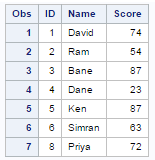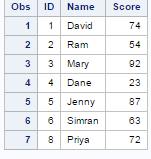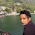# SAS : First. and Last. Variables

This tutorial explains how to identify first and last observations within a group. It is a common data cleaning challenge to remove duplicates or store unique values. In SQL, we use window functions such as rank over() to generate serial numbers among a group of rows. In SAS, we can create first. and last. variables to achieve this task.

First. and Last. Variables

FIRST.VARIABLE assigns the value of 1 for the first observation in a BY group and the value of 0 for all other observations in the BY group.
LAST.VARIABLE assigns the value of 1 for the last observation in a BY group and the value of 0 for all other observations in the BY group.
Note : Data set must be sorted BY group before applying FIRST. and LAST. Variables.

SAMPLE DATA SET

Suppose you have a dataset consisting 3 variables and 12 observations. The variables are ID, Name and Score. The variable ID is a grouping variable and it contains duplicates.

ID Name Score
1 David 45
1 David 74
2 Sam 45
2 Ram 54
3 Bane 87
3 Mary 92
3 Bane 87
4 Dane 23
5 Jenny 87
5 Ken 87
6 Simran 63
8 Priya 72

Create this data set in SAS

The program below creates the dataset in SAS. Copy the program below and paste it into SAS program editor and run/submit it.

Use PROC SORT to sort the data set by ID. It is required to sort the data before using first. and last. variables.

Note : FIRST./LAST. variables are temporary variables. That means they are not visible in the newly created data set. To make them visible, we need to create two new variables. In the program above, i have created First_ID and Last_ID variables.SAS : FIRST. vs. LAST. Variables
How it works

FIRST.variable = 1 when an observation is the first observation in each group values of variable ID.
FIRST.variable = 0 when an observation is not the first observation in each group values of variable ID.

LAST.variable = 1 when an observation is the last observation in each group values of variable ID.
LAST.variable = 0 when an observation is not the last observation in each group values of variable ID.
When FIRST.variable = 1 and LAST.VARIABLE = 1, it means there is only a single value in the group. (See ID = 4 in the above data for reference)

Selecting First Observation within a Group

Suppose you need to select only the first observation among a group of observations. It is very easy to do it with IF statement. The IF statement subsets data when IF is not used in conjunction with THEN or ELSE statements.
BY ID;
RUN;
BY ID;
IF FIRST.ID;
PROC PRINT;
RUN;Output : First. Variable

Note : It returns first observation among values of a group (total 7 observations).

Selecting Last Observation within a Group

Suppose you are asked to include only last observation from a group. Like the previous example, we can use last. variable to subset data.
BY ID;
RUN;
BY ID;
IF LAST.ID;
PROC PRINT;
RUN;SAS : Last. Variable

Q. Can we use WHERE instead of IF with First. and Last. Variables?
No. WHERE statement cannot be used with First. and Last. Variables. It is because WHERE statement requires variables already be created in the dataset before processing.

Generate Serial Number in a Group

Suppose you need to create serial numbers among a group of observations. See the snapshot below -Generate Serial Number in a Group
Data temp;
by ID;
if first.id then N = 1;
else N +1;
proc print;
run;
In the above program, we are setting N=1 when it is the first value of a group i.e. ID. Otherwise adding 1 to N. The N+1 implies N = N + 1 in BY group processing. When there is a second observation in a group, N+1 adds 1 to N=1 so N becomes 2. It further increments by 1 when there is third observation in the group and so on.

Calculate Cumulative Score by Group

Suppose you need to calculate running cumulative score by variable ID.Cumulative Score by Group
Data temp;
by ID;
if first.id then CumScore = Score;
else CumScore + Score;
proc print;
run;
In the above program, we are setting Cumscore = Score when it is the first value of a group i.e. ID. Otherwise adding Score to Cumscore. The Cumscore+Score implies CumScore = CumScore + Score in BY group processing.

STORING UNIQUE AND DUPLICATE VALUES
data unique duplicates;
by id;
if first.id = 1 and last.id = 1 then output unique;
else output duplicates;
run;
-The DATA statement creates two temporary SAS data sets: DUPLICATES AND UNIQUE.

-The BY statement tells SAS to process observations by ID. Variables FIRST.ID and LAST.ID are created.

-If the first and last observation is the same value, it implies it is a unique value else the value is duplicate.

Case Studies

1. Identify and select only records having maximum Score among a group of observations of variable ID

2. Select unique observations plus second observation from duplicate observations of variable ID

Solution 1

First we need to build a logic how we can select records having max score within variable ID. We can do it via PROC SORT. In this case, we need to sort data by 2 variables - first sorting on variable ID and then next sorting on Score by descending order. The DESCENDING keyword is used in PROC SORT to arrange data from largest to smallest. Sorting on descending order is used to place the max value at first observation in each group of ID.
by ID descending score;
run;

by ID;
if first.id;
run;
After sorting, we retain records having maximum value by using FIRST. and IF statement. The IF  FIRST.ID keeps the first record among a group of values of variable ID. The output is shown in the image below.Output
Solve second case study yourself and post your answer in the comment box below. Make sure it should be solved in one data step code. Hint : BOTH FIRST. and LAST. variables would be used.Related PostsDeepanshu founded ListenData with a simple objective - Make analytics easy to understand and follow. He has over 8 years of experience in data science. During his tenure, he has worked with global clients in various domains like Banking, Insurance, Telecom and Human Resource.

49 Responses to "SAS : First. and Last. Variables"
1.nice explaination

2.good explanation and good examples

3.data test8;
by ID;
if first.ID =1 and last.ID = 1 then output test8;
else if mod(_n_,2) = 0 then output test8;
run;
proc print; run;

1.data uniq_or_Dup2;
if (first.Id=1 and last.id=1) or (lag1(first.id)=1 and first.id=0) then output uniq_or_Dup2;
proc print
run;

2.This comment has been removed by the author.

4.proc sort data=data;
by ID;
run;

data data1;
set data;
by ID;
if first.ID=1 then serial=1;
else serial+1;
run;

data data2;
set data1;
by id;
if first.ID=1 and last.ID=1 or serial=2;
run;

1.good one..thanks

5.if we have four duplicates how can we get second one ?

1.first ne 1 and last. ne 1;

6.data test8;
by ID;
if first.ID =1 and last.ID = 1 then output test8;
if first.id and last.id=0 then N=1;
else N+1;
if N=2 then output test8;
drop N;
run;

7.data nk;
by id;
if first.id then N=1;
else N+1;
if N=2 then output;
if first.id and last.id then output;
drop N;
proc print;
run;

8.data new2;
retain cnt;
if first_id=1 then cnt=1;
else cnt+1;
if cnt le 2;
run;

1.this is the best logic ,i guess. you forgot to add by statement. i am just starter and took time to findout that ...

2.rajeshmanepalli987@gmail.com,if you are comfortable and ready to help, I have some doubts adn will drop a mail to you.If you are intersted ,no problem. thanks.

9.set abcd_s;
by x;
if first.x and last.x then output;
if first.x then n=1;
else n+1;
if n=2 then output;
drop n;
run;

10.by id;
if first.id then n =1;
Else n+1;
if first.id = 1 and last.id= 1 or n = 2;
drop n
Run;

11.Its very helpful, I have come across many questions in my interviews

12.data gaurav;
by id;
if first.id then N=1;
else N+1;
if (first.id=1 and last.id=1) then output;/* unique observation*/
if N=2 then output;/* second observation from duplicate*/
run;
proc print;
run;

13.If we have 4 or more than 4 duplicate ids then what would be the logic to find out 2nd observation?
Thanks!

14.by ID descending score;
run;

by ID;
if last.id;
run;

15.if i want to calculate average per person then how to calculate??

1.by ID Score;
run;

data temp;
by ID;
if first.ID then
do;
N=1;
cumScore = Score;
avgScore = Score;
end;
else
do;
N+1;
cumScore + Score;
avgScore = cumScore/N;
end;
if last.ID = 1;

run;

16.Doesn't generating serial numbers as well as cumulative rank require the newly created variables - N and Cum Score to be retained. Else, SAS will not be able to store their values jumping from one obs to the next ?

17.Below code is working for case study 2. Let me know if anybody still facing any problem in solving or understanding this question.

BY ID Descending score;
run;

BY ID ;
First_ID= First.ID;
Last_ID= Last.ID;
IF first.id then N=1;
ELSE N+1;
if (FIRST_ID=1 and LAST_ID=1) or N=2 then output READIN2 ;
drop First_ID Last_ID N;
proc print;
run;

1.18.How can we use ELSE N+1 in data step with using retain? Data step works obs by obs din't N loses its previous value in every next iteration?
I know, I am missing something, but what?

1.Retain can be used in two ways:
1. Implicitly : We can use retain implicitly by using +1 notation. If we use retain implicitly then we don't need to write retain statement. just like above code.
Another example :
data a;
set xyz;
by id;
if first.id then x=1;
else x+1 : /which means x=x+1 but we are using retain implicitly so no need to mention x= here;
run;

2. Explicitly

Using the same example where we are using retain explicitly so need to mention retain statement.
data a;
set xyz;
by id;
retain x;
if first.id then x=1;
else x=x+1 : /here we are using x= so need to mention retain statement;
run;

19.This comment has been removed by the author.

20.proc sort data=test;
by id score;
run;

set test;
by id score;
first_var=first.id;
last_var=last.id;
if (first_var=1 and last_var=1) or last_var=1;
run;

21.proc sort data=test;
by id;
run;

set test;
by id;
if (first.id=1 and last.id=1) then output unique;
if (last.id=1) then output second_dup;
run
proc print;
run;

1."if (last.id=1) then output second_dup;" will give unique records as well.
moreover if we have more than 2 records for for any ID then it will give last record for that and not the second record.
So this will work only for those IDs which are having exactly 2 records only.
It will be better if you can assign serial number by grouping on ID basis and then select value for that serial no=2 for 2nd observation.
Please let me know if you are having any confusion at any point.

22.by id;
if first.id=1 and last.id=1 then serial=1;
else serial+1 ;
run;

where serial=1 or serial=2;
run;

23.This comment has been removed by the author.

24.data aaa;
by id;
if first.id then n=1;
else n+1;
if not (first.id and last.id) and n<=2;
run;

25.data unique (drop =N) second (drop =N);

by id;
retain N;
if first.id =1 and last.id =1 then output unique ;
if first.id then N= 1;
else N= N+1;
if N =2 then output second;

run;

26.BY ID;
RUN;
DATA TEMP;
BY ID;
IF FIRST.ID=1 AND LAST.ID=1 THEN OUTPUT TEMP;
ELSE IF MOD(_N_,2)=0 THEN OUTPUT TEMP;
RUN;
PROC PRINT;
RUN;

27.Hi All, With refer to below dataset, Can anyone please assist me I want to have all those Empid's who have done more then 5 Transactions

data temp;
input EMPID Expenses;
CARDS;
101 200
102 300
103 200
104 500
105 600
101 450
102 600
101 400
101 200
101 700
102 600
101 800
101 500
102 900
101 500
102 600
102 800
102 600
102 900
102 700
102 600
102 600
103 800
;

1.someone please try to give solution for this query.

28.29.how to give the serial number to the dates by patients wise ....

Pt Date want in Days or want as serial
43 10/9/2018 DAY 1 1
43 10/10/2018 DAY 2 2
43 10/11/2018 DAY 3 3
43 10/12/2018 DAY 4 4
43 10/13/2018 DAY 5 5
43 10/15/2018 DAY 7 7
44 10/9/2018 DAY 1 1
44 10/11/2018 DAY 3 3
45 10/9/2018 DAY 1 1
45 10/10/2018 DAY 2 2
45 10/12/2018 DAY 4 4

30.given like this ,,...
Pt Date
43 10/9/2018
43 10/10/2018
43 10/11/2018
43 10/12/2018
43 10/13/2018
43 10/15/2018
44 10/9/2018
44 10/11/2018
45 10/9/2018
45 10/10/2018
45 10/12/2018

31.and convert as the
Pt want in Days or want as serial
43 DAY 1 1
43 DAY 2 2
43 DAY 3 3
43 DAY 4 4
43 DAY 5 5
43 DAY 7 7
44 DAY 1 1
44 DAY 3 3
45 DAY 1 1
45 DAY 2 2
45 DAY 4 4
in SAS

32.by id;
run;

data unique duplicate;
if first.id=1 and last.id=1 then output unique;
else output duplicate;
run;

proc sort data= duplicate;
by id;
run;

data duplicate;
set duplicate;
by id;
if first.id;
run;

33.If we want to select every third observations from a group then how we can get this. Note: we can't sort this because we don't want every third after sorting we want the third one from every group as it is.

34.BY ID ; RUN;
BY ID;
IF (FIRST.ID=0 AND LAST.ID=1) OR (FIRST.ID=1 AND LAST.ID=1);
RUN;

35.BY ID;
RUN;
/*DATA UNIQUE SECOBS;*/
DATA OBS;
BY ID DESCENDING SCORE;
/*IF FIRST.ID=1 AND LAST.ID=1 THEN OUTPUT UNIQUE;*/
IF FIRST.ID=1 AND LAST.ID=1 THEN OUTPUT OBS;
/*ELSE IF FIRST.ID=0 AND LAST.ID=1 THEN OUTPUT SECOBS;*/
ELSE IF FIRST.ID=0 AND LAST.ID=1 THEN OUTPUT OBS;
PROC PRINT;
RUN;
PROC PRINT DATA=UNIQUE;
RUN;
PROC PRINT DATA=SECOBS;
RUN;

36.Hi need some help

id var1 var2
1 20 30
1 40 50
1 30 55
2 22 45
2 37 49
3 79 36
4 36 49
4 68 78

I need to find the mean of var1 and var2 individually for the same ID
and fix it to the same ID variable

for example for ID 1 var1=mean(20,40,30) var2=mean(30,50,55)
and should show
ID Var1 Var2
1 30 45
2 29.5 47
.
.
.
Thank you

37.Data t;
Input x\$ y;
A 5
B 3
C 4
A 6
B 9
C 3
;
Run;

How to get out put
Sum of
A=
B=
C=

38.Hi All, With refer to below dataset, Can anyone please assist me I want to have all those Empid's who have done more then 5 Transactions

data temp;
input EMPID Expenses;
CARDS;
101 200
102 300
103 200
104 500
105 600
101 450
102 600
101 400
101 200
101 700
102 600
101 800
101 500
102 900
101 500
102 600
102 800
102 600
102 900
102 700
102 600
102 600
103 800
;

Next → ← Prev
Love this Post? Spread the Word!
Share